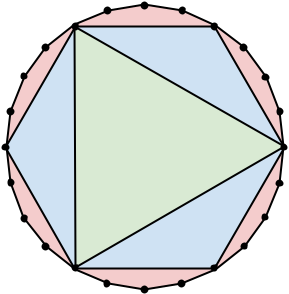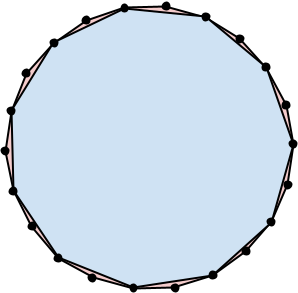시간 제한메모리 제한제출정답맞힌 사람정답 비율
서브태스크 참고 (추가 시간 없음) 1024 MB (추가 메모리 없음)47373485.000%

## 문제

A matryoshka is a type of doll that originated in Russia over a century ago. Their defining characteristic is that they consist of a set of dolls, all of a different size, with smaller dolls fitting nicely inside larger dolls.

In this problem, we work with matrygons, which are sets of regular convex polygons that follow a similar nesting pattern. A matrygon consists of a set of regular convex polygons with positive area p1, p2, …, pk such that, for all i, the vertices of pi+1 overlap with a proper subset of the vertices of pi (pi+1 has strictly less vertices than pi).

For example, the following pictures illustrates two matrygons. The first one contains 3 regular convex polygons: a regular icositetragon (24 sides), a regular hexagon (6 sides), and an equilateral triangle (3 sides). The second one contains 2 regular convex polygons: a regular icosidigon (22 sides) and a regular hendecagon (11 sides). Each of these matrygons has 33 total sides among all polygons in it.Given a fixed total number of sides N, calculate the largest number of polygons that can be part of a matrygon such that the total number of sides among all polygons in it is exactly N.

## 입력

The first line of the input gives the number of test cases, T. T lines follow. Each line represents a test case and contains a single integer N, the target total number of sides.

## 출력

For each test case, output one line containing Case #x: y, where x is the test case number (starting from 1) and y is the maximum number of polygons in a matrygon such that the total number of sides among all polygons in it is exactly N.

• 1 ≤ T ≤ 100.

## Test Set 1 (7점)

시간 제한: 20 초

• 3 ≤ N ≤ 1000.

시간 제한: 40 초

• 3 ≤ N ≤ 106.

## 예제 입력 1

3
33
15
41


## 예제 출력 1

Case #1: 3
Case #2: 2
Case #3: 1


## 힌트

The first matrygon pictured in the problem statement is an optimal solution for Sample Case #1.

In Sample Case #2, we can get to two polygons by fitting a regular pentagon (5 sides) inside a regular decagon (10 sides).

In Sample Case #3, there is no way to create a matrygon with multiple regular polygons, so our only option is to use a single regular tetracontahenagon (41 sides).

## 채점 및 기타 정보

• 예제는 채점하지 않는다.